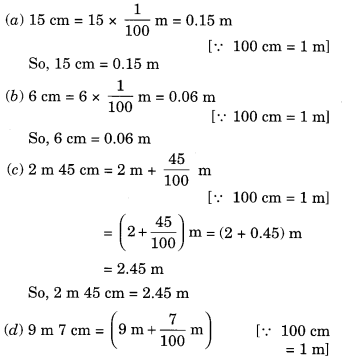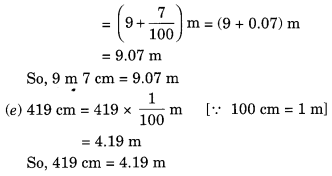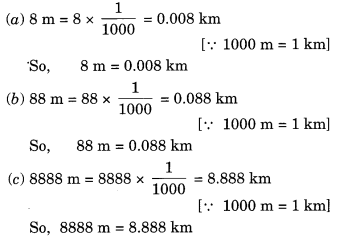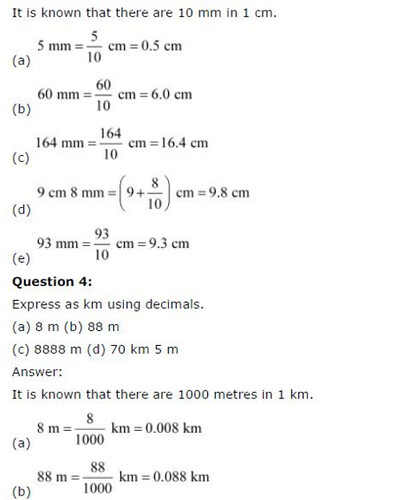# NCERT Solutions For Class 6 Maths Decimals Exercise 8.4

NCERT Solutions For Class 6 Maths Decimals Exercise 8.4

### NCERT Solutions For Class 6 Maths Decimals Exercise 8.4

NCERT Solutions for Class 6 Maths Chapter 8 Decimals Ex 8.4

Exercise 8.4

Ex 8.4 Class 6 Maths Question 1.
Express as rupees using decimals.
(a) 5 paise
(b) 75 paise
(c) 20 paise
(d) 50 rupees 90 paise
(e) 725 paise
Solution:= ₹7.25
So, 725 paise = ₹7.25

Ex 8.4 Class 6 Maths Question 2.
Express as metres using decimals,
(a) 15cm
(b) 6cm
(c) 2m 45cm
(d) 9m 7cm
(e) 419 cm
Solution:Ex 8.4 Class 6 Maths Question 3.
Express as cm using decimals.
(a) 5 mm
(b) 60 mm
(c) 164 mm
(d) 9 cm 8 mm
(e) 93 mm
Solution:Ex 8.4 Class 6 Maths Question 4.
Express as km using decimals.
(a) 8 m
(b) 88 m
(c) 8888 m
(d) 70km 5m
Solution:Ex 8.4 Class 6 Maths Question 5.
Express as kg using decimals.
(a) 2 g
(b) 100 g
(c) 3750 g
(d) 5 kg 8 g
(e) 26 kg 50 g
Solution:## SabDekho

The Complete Educational Website